Libri di Francesca Greselin - libri Statistica & Applicazioni

# Francesca Greselin

### Titoli dell'autore

Socio-economic evaluation with ordinal variables: integrating counting and poset approaches
Free
digital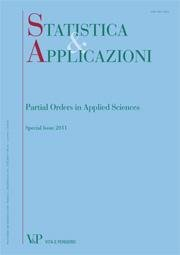Year: 2011
The evaluation of material deprivation, quality of life and well-being very often requires to deal with multidimensional systems of ordinal variables, rather than with classical numerical datasets. This poses new statistical and methodological challenges, since classical evaluation tools are not designed to deal with this kind of data. The mainstream evaluation methodologies generally follow a counting approach, as in a recent proposal by Alkire and Foster pertaining to the evaluation of multidimensional poverty. Counting procedures are inspired by the composite indicator approach and share similar drawbacks with it, computing aggregated indicators that may be poorly reliable. A recent and alternative proposal is to address the ordinal evaluation problem through partial order theory which provides tools that prove more consistent with the discrete nature of the data. The goal of the present paper is thus to introduce the two proposals, showing how the evaluation methodology based on partial order theory can be integrated in the counting approach of Alkire and Foster. Keywords: Partial Order theory, Counting Approach, Evaluation, Material Deprivation, Quality of Life
Minimum sample sizes in asymptotic confidence intervals for Gini’s inequality measure
Free
digital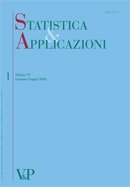Year: 2008
Statistical inference for inequality measures has been of considerable interest in recent years. Income studies often deal with very large samples, hence precision would not seem a serious issue. Yet, in many empirical studies large standard errors are observed (Maasoumi, 1997). Therefore, it is important to provide methodologies to assess whether differences in estimates are statistically significant. This paper presents an analysis of the performance of asymptotic confidence intervals for Gini’s index, virtually the most widely used inequality index. To determine minimum sample sizes assuring a given accuracy in confidence intervals, an extensive simulation study has been carried out. A wide set of underlying distributions has been considered, choosing from specific models for income data. As expected, the minimum sample sizes are seriously affected by some population characteristics as tail heaviness and asymmetry. However, in a wide range of cases, it turns out that they are smaller than sample sizes actually used in social sciences.
Minimum sample sizes for confidence intervals for Gini’s mean difference: a new approach for their determination
Free
digital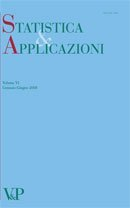Year: 2007
The sample mean difference Â is an unbiased estimator of Gini’s mean difference A. It is well known that Â is asymptotically normally distributed (Hoeffding, 1948). In order to obtain confidence intervals for A, Â must be standardized and hence its variance Var(Â) must be estimated. In this paper we study the effective coverage of the confidence intervals for A, when using a specific unbiased estimator ^ Var(Â) for the variance of Â, in a non-parametric framework. The empirical determination of the minimum sample size required to reach a good approximation of the nominal coverage is analyzed through a new approach. The reported results show that this threshold is critically related to the asymmetry and the tail heaviness in the underlying distribution.
Convergence of the Sample Mean Difference to the normal distribution: simulation results digitalYear: 2006
The present work aims to obtain the value of minimum sample size required by a good approximation by the normal curve for the sample mean difference. Particular care is given to what happens in the tails of the curves, with the aim of deriving confidence intervals for Gini’s mean difference. This goal is obtained by empirical methods and the presented results have an explorative nature. Simulation data have been obtained sampling from different distributions, considering symmetry versus asymmetry and the existence of the moments as main aspects in the underlying distribution. These remarks lead to the choice of the normal, the rectangular, the exponential and the Pareto distributions. All the obtained results indicate that the shape of the distribution from which the samples are generated is critically related to the minimum sample sizes required for a good approximation of the tails of the sample mean difference to the normal curve. Keywords: Gini Mean Difference, asymptotic distribution, convergence, U-statistic.
Dependence measures based on partial and total orderings digital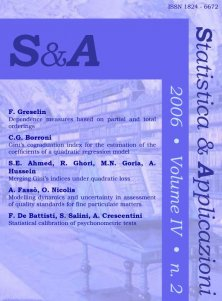Year: 2006
The aim of this paper is to propose a new operational measure for evaluating the degree of dependence existing between two nominal categorical variables.
Counting and enumerating frequency tables with given margins digital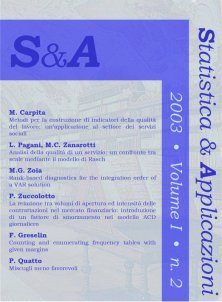Year: 2003
Consider the integral m × n table X whose entries are count data for a two-way classification scheme. We shall be concerned first with the problem of counting up, then of enumerating all contingency tables with given margins.